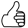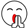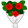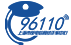• 最近访问：

(海润光伏巨亏45亿)=(料想到暂停上市)=(要拖时间)=(假重组)=(掏光海润
(海润光伏巨亏45亿)=(料想到暂停上市)=(要拖时间)=(假重组)=(掏光海润所有资产)=(拖一年时间)=(退市)=(所有高管全逃跑)=(必须告)=(证监会已立案调查)=(孟广宝杨怀进是主谋)=(邱新问闻徐小平李延人是共犯)=(这些人必须全进监狱)

666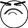666

6

，除了杨与张j

666

999

7